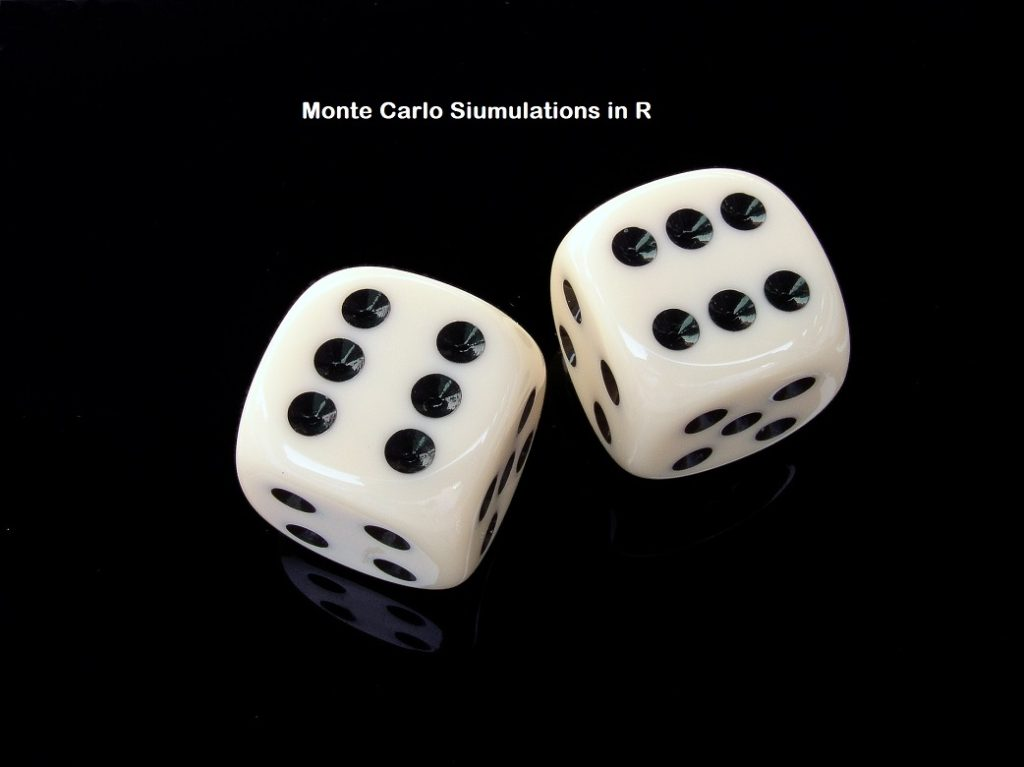# Monte Carlo Simulations in R

[This article was first published on R programming – Journey of Analytics, and kindly contributed to R-bloggers]. (You can report issue about the content on this page here)
Want to share your content on R-bloggers? click here if you have a blog, or here if you don't.

In today’s tutorial, we are going to learn how to implement Monte Carlo Simulations in R.

## Logic behind Monte Carlo:Monte Carlo Simulations in R

Monte Carlo simulation (also known as the Monte Carlo Method) is a statistical technique that allows us to compute all the possible outcomes of an event. This makes it extremely helpful in risk assessment and aids decision-making because we can predict the probability of extreme cases coming true. The technique was first used by scientists working on the atom bomb; it was named for Monte Carlo, the Monaco resort town renowned for its casinos. Since its introduction in World War II, Monte Carlo simulation has been used to model a variety of physical and conceptual systems.

Monte Carlo methods are used to identify the probability of an event A happening, among a set of N events. We assume that all the events are independent, and the probability of event A happening once does not prevent the occurrence again.

For example, assume you have a fair coin and you flip it once. The probability of heads is 0.5 i.e. equal possibility of heads or tails. You flip the coin again. The possibility of heads is still 0.5, irrespective of whether we got heads or tails in the first flip. However, we can safely say that if we were to flip the coin 100 times, you would see heads ~50% of the times. The application of Monte Carlo (referred henceforth in this post as MC) methods comes to play when we want to find out the probability of heads occurring 16 times in a row. (or 5 or 3 or any other number.)

2. MC methods in Finance, from Investopedia.com – link2

## Applications:

MC methods are used by professionals in numerous fields ranging from finance, project management, energy, manufacturing, R&D, insurance, biotech, etc. Some real-world applications of Monte Carlo simulations are given below:

1. Monte Carlo simulations are used in financial services to predict fraudulent credit card transactions. (since 100 genuine transactions do not guarantee the next one will not be fraudulent, even though it is a rare event by itself.)
2. Risk analysis. Assume a new product was sold at a loss of \$300 to 6 users (due to coupons or sales), a profit of \$467 in 79 users and a profit of \$82 to 119 customers. We can use Monte Carlo simulations to understand what would be the average P/L (profit or loss) if 1000 customers bought our products.
3. A/B testing to understand page bounce and success web elements. Assume you changed the payment processing system on your e-commerce site. You are doing an A/B test to see if the upgrade results in improved checkout completion. On the old system, 12 users abandoned their cart, while 19 completed their purchase. On the new system, 147 people abandoned their cart while 320 completed their purchase. Which system works better?
4. Selection criteria. Example if we have 7 candidates for a scholarship (Eileen, George, Taher, Ramesis, Arya, Sandra and Mike) what is the probability that Mike will be chosen in three consecutive years? Assuming the candidate list is the same and past winners are not barred from receiving the scholarship again.

Unlike simple forecasting, Monte Carlo simulation can help with the following:

• Probabilistic Results – show scenarios and how the occurrence likelihood.
• Graphical Results – The outcomes and their chance of occurring can be easily converted to graphs making it easy to communicate findings to an audience.
• Sensitivity Analysis – Easier to see which variables impact the outcome the most, i.e. which variables had the biggest effect on bottom-line results.
• Scenario Analysis: Using Monte Carlo simulation, we can see exactly which inputs had which values together when certain outcomes occurred.
• Correlation of Inputs. In Monte Carlo simulation, it’s possible to model interdependent relationships between input variables. It’s important for accuracy to represent how, in reality, when some factors goes up, others go up or down accordingly.

## Code template:

The basic template for MC is as follows:

```runs <- 100000
func1 <- sum(sample(c(0,1), size =10, replace = T)) > 6
mc_prob  <- sum(replicate(runs, func())) / runs```

Let’s look at this code in detail:

• Runs = no of trials or iterations. For our product profit example (application example 2), runs = 1000.
• Func1 = this is the formula definition where we will indicate number of different events, their probability and the selection criteria. For our scholarship candidate example (application number 4) this function would be modified as:

sum(sample(c(1:7), size =3, replace = T)) > 6

where we are assigning number 1:7 to each student and hence Mike = 7.

## Main code:

The code files for this tutorial are available on the 2017 project page. (Link here under Jul/Aug 2017 ) .

R-bloggers.com offers daily e-mail updates about R news and tutorials about learning R and many other topics. Click here if you're looking to post or find an R/data-science job.
Want to share your content on R-bloggers? click here if you have a blog, or here if you don't.